# Story

It has been a long time since we last wrote a post. A recent discussion that I was a part of sparked the impetus for this post. The attempt, as always, would be to simplify the discussion for an average reader to understand and appreciate, however, some elementary knowledge about regressions and time series will be implied in few parts of the discussions. We wrote a few posts earlier on stationarity, non-stationarity of timeseries and also briefly about time series regressions in general. It would be helpful for the reader to refer to some of these previous posts to acquaint him/her self with some basic concepts of timeseries to better follow the arguments in the post.

The focus of this post would be on multivariate timeseries modelling. There is fundamental difference in cross sectional and time series regression models, one most critical being the importance of sequence in timeseries. The sequence in which the data is analyzed is of significant importance in timeseries. Also, the dependence of relationships between variables is dependent on the lead or the lag values of the variables, which is unlike cross sectional regression analysis.

Most of the contemporary literature around multivariate timeseries modelling revolves around Vector Autoregression (VAR) models. This has garnered significant debate especially after Chris Sims shared a nobel prize in 2011 with Thomas Sargent for their empirical macroeconomic research which heavily revolved around use of VAR models. Even as the literature is evolving on VAR models, in abundant cases we find usage of simple OLS regression for timeseries modelling. I would leave the discussion around VAR models for another post and would discuss some key tenets of multivariate timeseries regression modelling in this post.

# Components of timeseries

Fundamentally speaking, there are 4 key components of any timeseries :

1. Trend component ($$T_t$$) - This is the deterministic or stochastic long term trend of the series, will discuss this below
2. Cyclical component($$C_t$$) - This is the fluctuations around the mean trend of the series
3. Seasonal component ($$S_t$$) - This is the systematic periodic predictable component of the series
4. Noise ($$\epsilon_t$$) - This is pure random noise

$Timeseries = T_t + C_t + S_t + \epsilon_t$

Each of these components of the timeseries need to be understood before one delves in the task of modelling. Its important to understand these components in order to build a robust statistical timeseries model and avoid capturing any spurious relationships in the model.

In most cases, what we are essentially trying to model in a timeseries is the “Cyclical” component. For modelling purpose it is an imperitive that the “cyclical” component of the series is decoupled with the rest of the components before the analysis. We will discuss why this is important in the following sections.

# Stationarity

The first and foremost property of the timeseries that needs to be looked at is stationarity. There are 3 forms of stationarity one can expect to find. Refer to these for a recap.

1. Stationary (or I(0)) - Mean and Variance remain constant over time
2. Non-stationary (or Integrated series or I(1) series) - Mean and/or variance changes with time, the series follows a random walk (in the literal sense) and there is, what we call, a stochastic trend.

3. Trend stationary - Mean has a deterministic trend over time and detrended series is stationary (Trend stationarity is that elusive form of stationarity which, in my humble opinion, does not get the attention that it deserves. Its often confused with non-stationarity which might lead to disasterous implications for ones statistical analysis.)

The best way to illustrate this by using an visual example. Below is an example of the 3 forms of stationarity.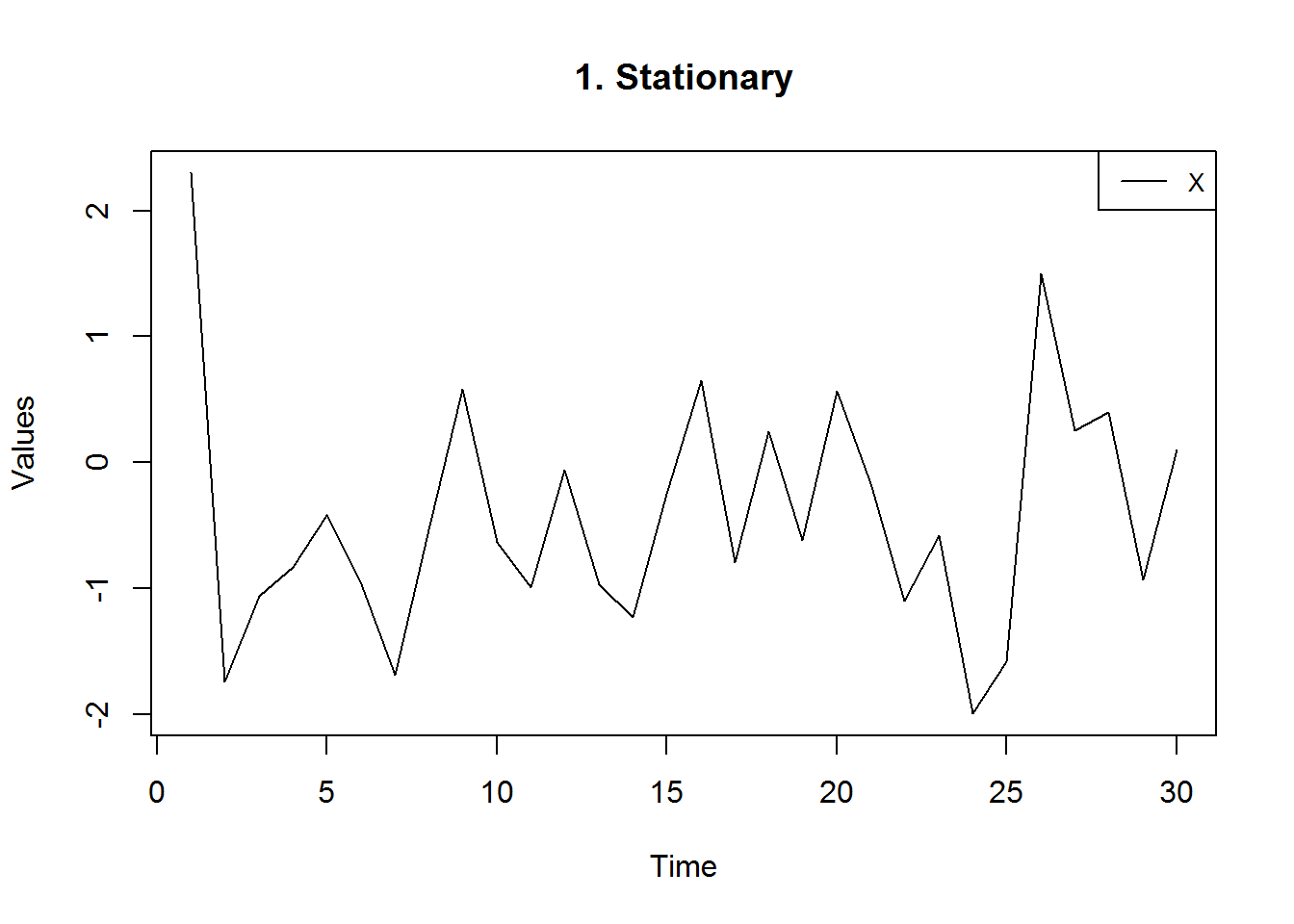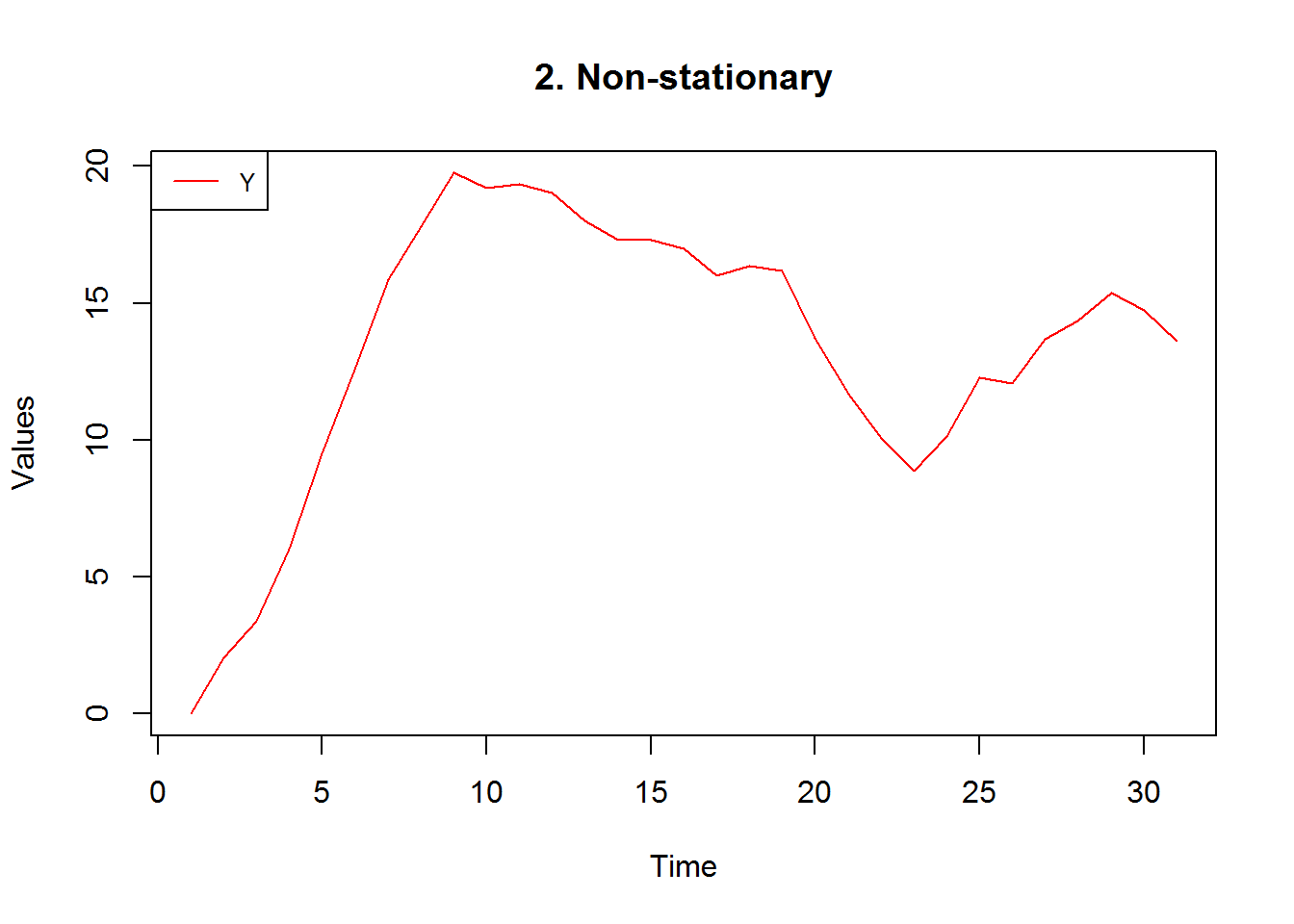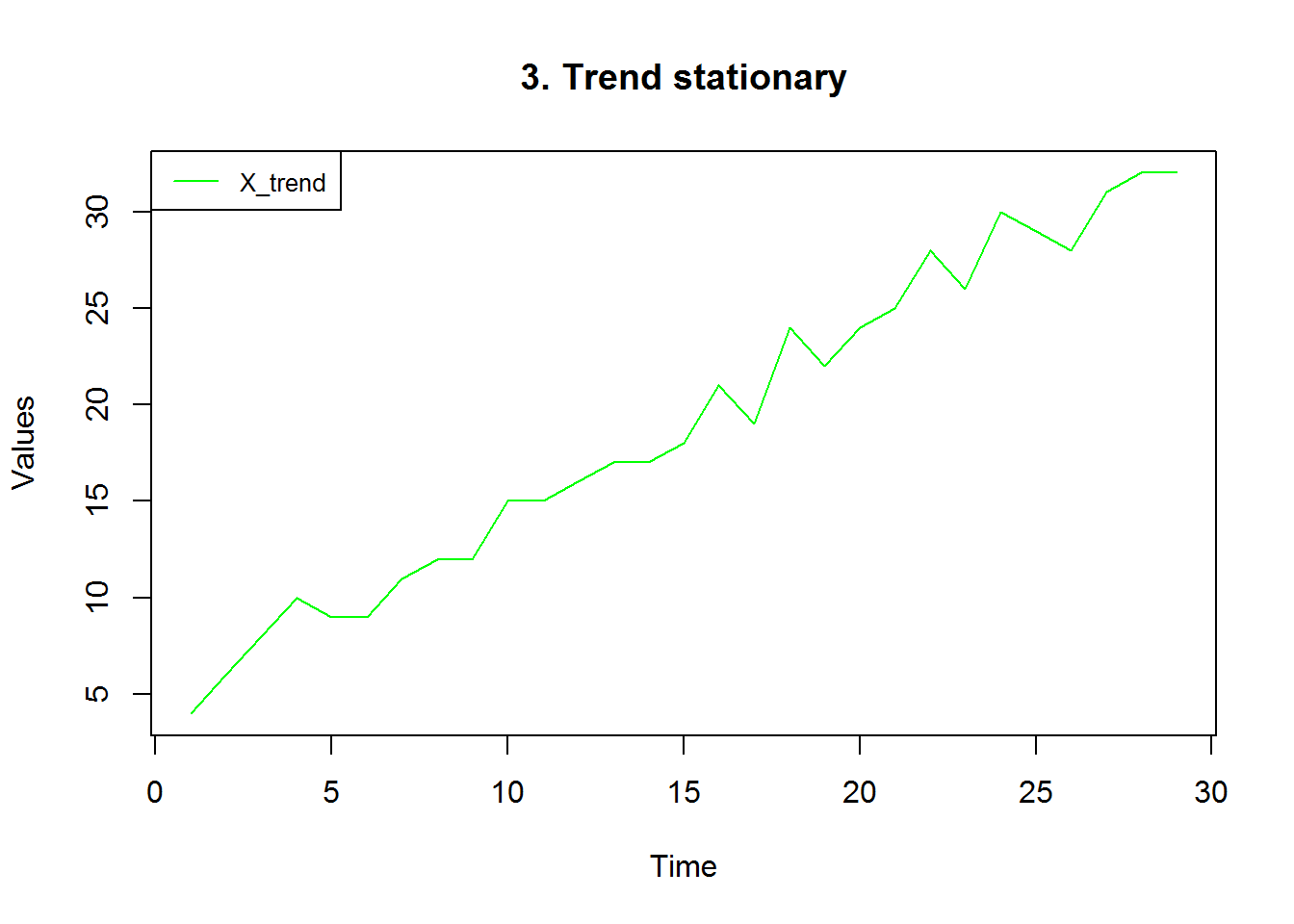# Two variable timeseries model

It is critical to first understand the nature of stationarity exhibited by both the series that one wishes to assess and based on the combination of relationships, transform the variables accordingly. Below table summarizes, in a nutshell, what needs to be done in the various combinations of relationships that one might expect between the 2 timeseries.

S.no Combination of relationship between the 2 variables Action to be performed before running regression
1 Both stationary No action required
2 Both trend stationary Detrend both series
3 Both Non stationary Co-integration check? (Discussed below)
4 One Stationary & one trend stationary Detrend the trend stationary process
5 One stationary & one non-stationary Difference the I(1) series
6 One trend stationary & one non-stationary Difference the I(1) series and detrend the trend stationary process

The reason these transformations are required is to ensure that in the modelling process, we are only focusing on the cyclical component. If you think about it, the rest of the part of the timeseries components are deterministic per say. Hence, what we “really” want to be looking at is whether the cyclical component of one series helps us explain the cyclical component of the other series. Any relationship established otherwise, could potentially be a spurious one.

The 2 cases in the above table highlighted in bold are the cases where, in my opinion, there is most scope of confusion and chance for error. We will take up an example to drive home this point, but before that a confusion regarding the definition of cointegration needs to be understood where practitioners are most susceptible to commit a mistake.

# Cointegration Definition

Two non-stationary ( or I(1)) series “X” and “Y” are said to be cointegrated if the regression of Y on X yields residuals that are stationary (I(0)). Another way to define co-integration would be to define it as the presence of a long term equillibrium relationship that exists between 2 non-stationary series.

An excellent definition in laymans language can be found from Edwin Chens blog here.

Now, the best way to explain the confusion that the definition of cointegration brings is to start by reading a reference on Wikipedia here . The point to focus on is the following :

If there are I(1) series on both sides of the regression relationship, then there is a possibility that you will get misleading results from running a regression. So now check for co-integration between all the I(1) series. If this holds, this is a guarantee that the regression results you get are not spurious.

The above statement coupled with the limited understanding on trend stationary processes could be a recipe for disaster. Its important that both the series being used for analysis are “I(1)” for the above assumption to work and it Does Not Hold for Trend Stationary series.

That said, lets move on to the example and see how the above statement could be misleading for trend stationary series.

# Both series are trend stationary

To make the example more interesting lets assume that we are talking about the Kingdom of Winterfell (for all GoT fans!), the variable “Y” is the Happiness index (HI) of Winterfell and variable “X” is the GDP of Winterfell.

Now lets first discuss the hypothesis around the relationship that we would expect. Increase in GDP is associated with increase in employment and increase in disposible income of Winterfell (since there is no technological regime change) and since the citizens of Winterfell are very materialistic this translates into more happiness. Hence, the relationship we expect GDP to have with happpiness index (HI) is a positive one.

Let us look at what the relationship looks like in a visual inspection.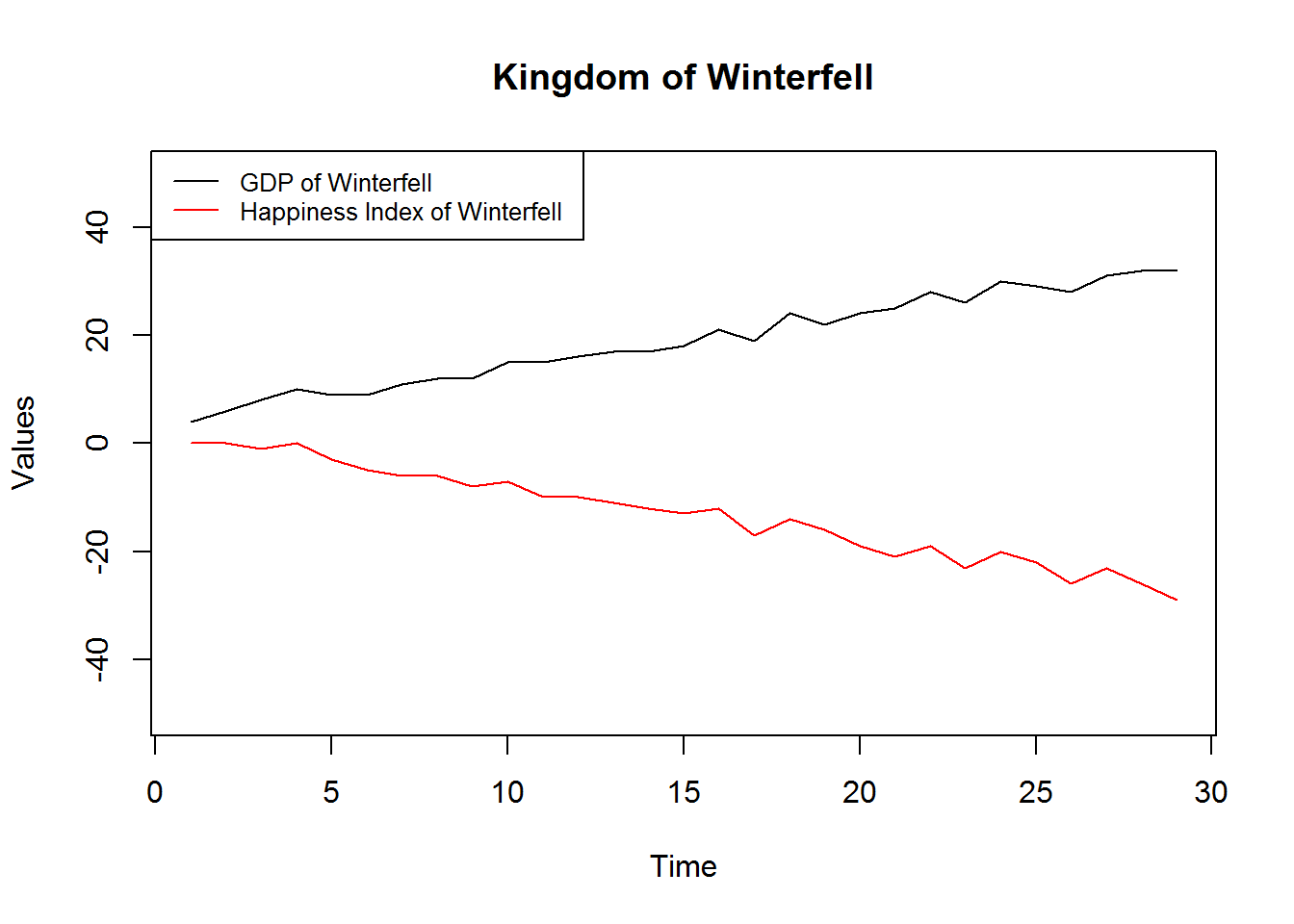From the graph above, we can infer that GDP and HI are both not stationary (but are trend stationary) and seem to have a long term relationship between them (which can be mistaken with cointegration), we could check the residuals of the regression of Happiness index (“Y”) on GDP (“X”) to see if they are stationary.

raw.result <- lm(Y ~ X, data = data)
adf.test(raw.result$residuals, k = 1) ## Warning in adf.test(raw.result$residuals, k = 1): p-value smaller than
## printed p-value
##
##  Augmented Dickey-Fuller Test
##
## data:  raw.result$residuals ## Dickey-Fuller = -5.5942, Lag order = 1, p-value = 0.01 ## alternative hypothesis: stationary  The adf.test on the residuals of the regression too suggests that the residuals of the regression are stationary (wrongly) suggesting that the series could be co-integrated. This is a common mistake that one might commit. As much as one would want to guess based on a visual glance, the 2 series are Not Cointegrated for the simple reason that they are not I(1), they are trend stationary. Cointegration could only result in non-stationary I(1) series and it is certainly not a property that can be eyeballed and inferred from a graph. There is a difference in the “deterministic” trend to be common (which is the case in our example), and the “stochastic” trend to be common (which is the case in cointegration). However, based on the impression of cointegration, one might be tempted to run the regression on the raw series. Lets see what that regression would reveal. ## ## Call: ## lm(formula = Y ~ X, data = data) ## ## Residuals: ## Min 1Q Median 3Q Max ## -4.0838 -1.5863 0.0405 1.3308 4.2893 ## ## Coefficients: ## Estimate Std. Error t value Pr(>|t|) ## (Intercept) 5.5034 1.0712 5.138 2.1e-05 *** ## X -0.9793 0.0517 -18.943 < 2e-16 *** ## --- ## Signif. codes: 0 '***' 0.001 '**' 0.01 '*' 0.05 '.' 0.1 ' ' 1 ## ## Residual standard error: 2.324 on 27 degrees of freedom ## Multiple R-squared: 0.93, Adjusted R-squared: 0.9274 ## F-statistic: 358.8 on 1 and 27 DF, p-value: < 2.2e-16 Well, the results have to say something which is completely contrary to what our hypothesis was. Lets look at why this would be happening. By eyeballing the plot above, it is clear why there is a negative relationship between the 2 series. There have been significant atrocities committed on the Starks of Winterfell, which explains the negative trend of the happiness index. And due to limited kingdom intervention in trade and the war that Rob Stark started, the GDP has been boosting over the past few years, which explains the positive trend in the series. Let’s take a step back and assess what we have done above. We have not decoupled the “Deterministic trend” and the “Cyclical” component of the series and due to that the relationships that we observe in the regression are purely driven by the trend (deterministic) component and not the cyclical component at all. If we were to actually test the relationship between the GDP of Winterfell and Happiness index, we would have to decouple the 2 components in the series and then run the regression model. # Detrending X (HI) reg.de.trend <- lm(X ~ t, data = data) data$Detrend_X <- reg.de.trend$residuals adf.test(reg.de.trend$residuals, k = 1) # Checking that the detrended component are now stationary
##
##  Augmented Dickey-Fuller Test
##
## data:  reg.de.trend$residuals ## Dickey-Fuller = -4.2817, Lag order = 1, p-value = 0.01252 ## alternative hypothesis: stationary # Detrending Y (GDP) reg.de.trend.Y <- lm(Y ~ t, data = data) data$Detrend_Y <- reg.de.trend.Y$residuals adf.test(reg.de.trend.Y$residuals, k = 1) # Checking that the detrended component are now stationary
## Warning in adf.test(reg.de.trend.Y$residuals, k = 1): p-value smaller than ## printed p-value ## ## Augmented Dickey-Fuller Test ## ## data: reg.de.trend.Y$residuals
## Dickey-Fuller = -5.264, Lag order = 1, p-value = 0.01
## alternative hypothesis: stationary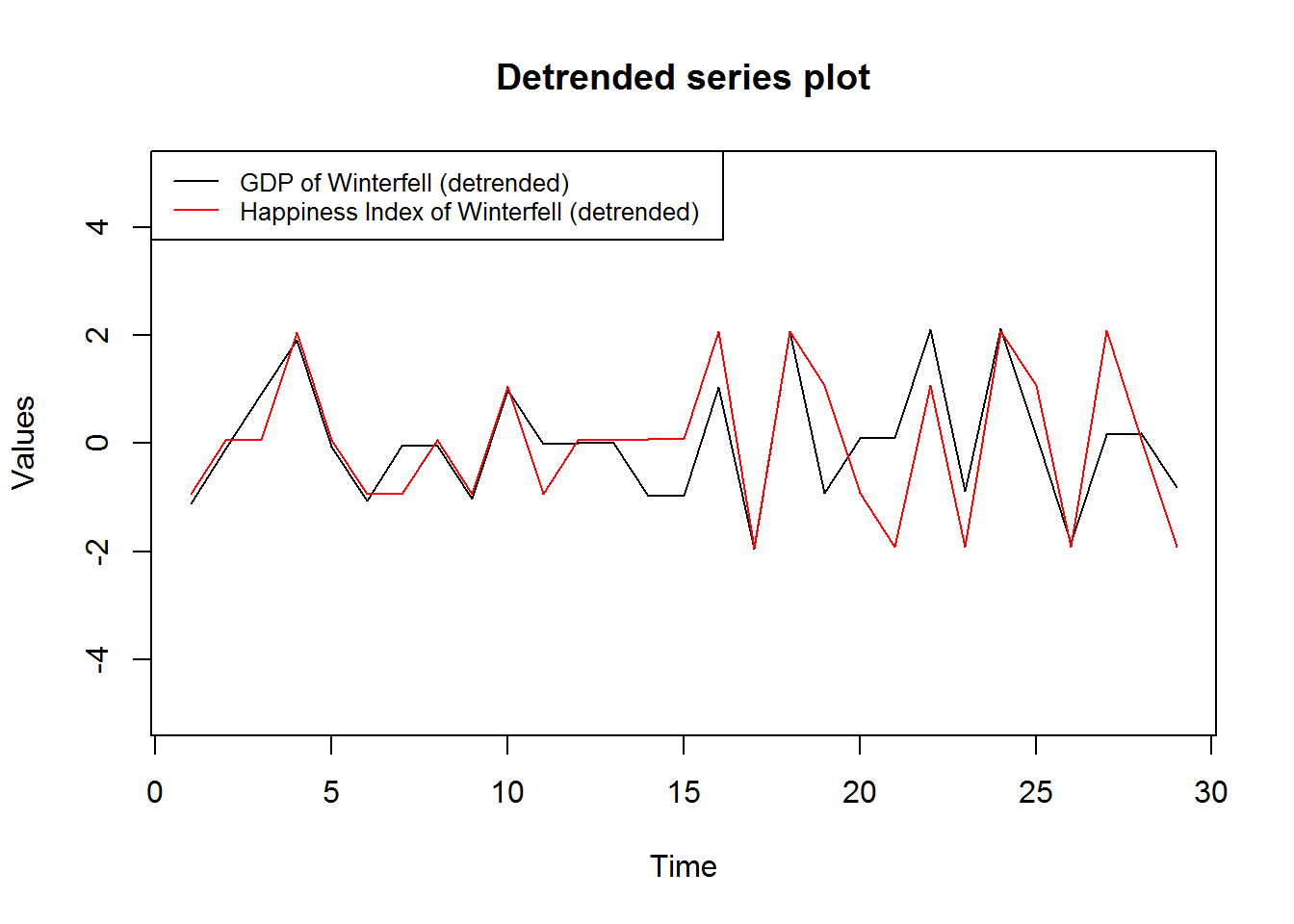Now, running regression with the de-trended components.

detrend.reg <- lm(Detrend_Y ~ Detrend_X, data = data)
summary(detrend.reg)
##
## Call:
## lm(formula = Detrend_Y ~ Detrend_X, data = data)
##
## Residuals:
##     Min      1Q  Median      3Q     Max
## -2.0137 -0.7479  0.0556  0.2322  1.9343
##
## Coefficients:
##              Estimate Std. Error t value Pr(>|t|)
## (Intercept) 2.383e-16  1.689e-01   0.000        1
## Detrend_X   8.901e-01  1.544e-01   5.765 3.94e-06 ***
## ---
## Signif. codes:  0 '***' 0.001 '**' 0.01 '*' 0.05 '.' 0.1 ' ' 1
##
## Residual standard error: 0.9098 on 27 degrees of freedom
## Multiple R-squared:  0.5518, Adjusted R-squared:  0.5352
## F-statistic: 33.24 on 1 and 27 DF,  p-value: 3.937e-06

Voila! The sign of the coefficients reversed implying that GDP does have a positive impact on the happiness index of Winterfell. This is a clear example of how the relationships that one arrives at are spurious if the 2 components of time series are not decoupled before running the regression.

Corollary : When dealing with 2 trend-stationary series in a regression model, always decouple the trend and cyclical component to avoid arriving are incorrect conclusions.

In the next post, we would discuss what the course of action should be in case both the series are non-stationary (or I(1)) and critique on the correct way to establish relationships in a regression model.

Feedback/criticisms welcome.

1.very helpful ! thank you for the concise demonstration.

2.Where did the 't' in the lm(X~t,data=data) line come from? I'm assuming this is for trend but lm doesn't recognize trend as a formal argument usually. The tslm function does but it doesn't work with the rest of your code. Can you perhaps provide a sample of the data you are using (head(data)) and please clarify your code a bit more?
I'm finding your text and example very useful but am missing a few key pieces.

Thanks,

RTJ

1.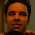You are right RTJ, apologies for not providing that part of the code in the blogpost. The "t" was explicitly created in the data.frame using the following code:

data\$t <- seq(from = 1, to = nrow(data), by = 1)

Once you create this "t" you can use that in the regression. I will update the post with this part of the code. Thanks for pointing this out!

~
Shreyes

3.hey! thanks for your post! i am an absolute beginner regarding regression etc. I have to do some analysis in SPSS and I have some major questions: I have a time-series cross-sectional dataset. i have data collected over 3 years for 10 different countries. my dependent variable is HAPPINESS and my ind. variables are income, health and religion. I want to see how happiness has changed over the years and for the different countries. how can I compare them? could i run regressions for each country and each year separatly and then put the numbers in one graph? that would probably be too simple. it would be so AMAZING if you could give me some advise!!

4.Hey:-), I am beginner and found your post quite useful for me. I really did great job. I found your text and example very useful. Keep it up!